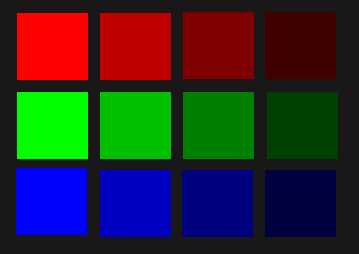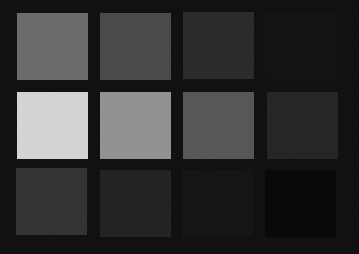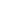### Formulas for Calculating Colour Brightness

Steps on the Way. Getting There.
A formula for determining whether text of a given colour or shade is readable over a background of a different colour or shade.
I am not a mathematician, I am essentially an artist, and also a computer programer, hence much of what I have discovered about this has been through trial and error. Here are the results of my current researches.
Non-linear
First of all, a computer monitor (and any type of display screen) does not get brighter linearly, the distance between two brightnesses of a given hue will be relatively greater, the brighter the colours are. For a knowledgeable explanation of this, see Frequently Asked Questions about Gamma by Charles Poynton.
This means that any formula that gives constants as factors to multiply out by, where you multiply the red, green and blue elements of the RGB triad each by a number to get a brightness value, e.g. formulas such as those in the W3C Guidelines, won’t work when you are wanting to compare two brightnesses. They can’t, and they don’t.
Photoshop Seems to Get it Right at First Sight
Photoshop gets it right. Or does it? Those greyscales you see in the examples below look right. So what formula does Photoshop use?
Here is a block produced in Photoshop showing red, green and blue at values 255, 192, 128 and 64:Now we convert that to greyscale and see what grey values Photoshop gives us.That looks pretty good perceptually. It goes like this:
 Colour value Red Green Blue 255 130 220 70 192 96 165 50 128 62 110 30 64 28 54 10
I don’t know for sure what formula Photoshop uses as I’m not privy to Photoshop’s internal code, but I do know of a formula that gives exactly the same greyscale results as Photoshop, and I know of another that is simpler and more rough-and-ready and which gives results that are pretty close to Photoshop’s. (Tested against Photoshop 5.5 thru CC, the greyscale conversion seems to have stayed consistent across the versions).
The formula for exactly equivalent results to Photoshop uses a gamma calculation. I got this code from StackOverflow, expressed there in C and I give it below in Javascript. As I explain below, these functions use classic sRGB gamma formulas.
As with all the various formulas for obtaining a greyscale, the like-Photoshop formula uses percentage values to multiply the red (R), green (G) and blue (B) numeric levels by. (see Puzzling Greys). The like-Photoshop formula uses R=0.2235, G=0.7154, B=0.0611 to get a result equivalent to what you get with Photoshop.
I say in the title to this section that Photoshop seems to get it right at first sight. It does so far as the examples on the left show, but as I explain below Photoshop gives a linear result for greyscale, which is not perfect for comparing the brightness levels of two shades of grey. Read on . . .
So, to the formulas:
The like-Photoshop Formula
First some functions in Javascript:
/* Inverse of sRGB "gamma" function. ********************** */
function inv_gam_sRGB( ic) {
var c = ic/255.0;
return c <= 0.04045 ? c/12.92 : Math.pow(((c+0.055)/(1.055)),2.4);
}
/* sRGB "gamma" function ******************************** */
function gam_sRGB(vi) {
var v=vi<=0.0031308 ? vi * 12.92 : 1.055*Math.pow(vi,1.0/2.4)-0.055
return (v*255);
}
/* GRAY VALUE ("brightness") *************************** */
function gamma_grey(r, g, b, rY, gY, bY) {
return gam_sRGB( rY*inv_gam_sRGB(r) + gY*inv_gam_sRGB(g) + bY*inv_gam_sRGB(b) );
}
You get a brightness (i.e. greyscale) value by calling:
brightness_value = gamma_grey(R, G, B, 0.2235, 0.7154, 0.0611);
Where R, G and B are the colour values of red, green and blue, of course.
You’ll find that gives you a brightness value exactly equivalent to Photoshop’s (well I do).
What the Formula is Doing
The formulas use a classic linear to sRGB and sRGB to linear conversion. Mathematically that can be seen on the page A close look at the sRGB formula and in structural code on Optimizing conversion between sRGB and linear.
The two Javascript functions shown above, gam_sRGB and inv_gam_sRGB are the inverse of one another, a colour value passed through gam_sRGB and its result through inv_gam_sRGB, or vice-versa, will give you back the colour value. To be more precise, if you use these functions standalone, you have to divide the colour value by 255 before passing it as a parameter to gam_sRGB – note that inv_gam_sRGB does that division as its first operation, whereas gam_sRGB doesn’t.
The gamma_grey function passes the colour value, R, G or B, though the inverse gamma sRGB inv_gam_sRGB and then multiplies the result by the R, G, or B constant factor. It then adds the R, G and B results from inv_gam_sRGB together and passes the result though the gamma sRGB gam_sRGB function to get a greyscale.
inv_gam_sRGB will always give a result from 0 to 1. This fraction is then multiplied by the factor for each of the R, G and B values.
Although these are gamma functions, the resulting greyscale will in effect be linear, that is because the colour values go through both gam_sRGB and inv_gam_sRGB, which has a cancelling-out outcome, see my page Colour Brightness Experiment which shows that, without further adjustment, a brightness range for a given colour triad is linear.
Values 0-255 calculated as sRGB give a curve, which is said to be very useful for adjusting colours on photographs, but it is a curve that it inverse to what we need when comparing two brightnesses. The sRGB curve with an exponent of 2.4 looks like this:
With this curve the brightnesses get less the higher the colour value, not more, so we need the inverse of this:
That is the chart for 0-255 through the inv_gam_sRGB formula.
Similar but Rougher
You can get almost identical results, slightly further out in the low values values, by forgetting all about complex gamma calculations and using something much simpler.
brightness_value = (0.22475*R^2.235 + 0.7154*G^2.235 + 0.05575*B^2.235)^(1/2.235)
Thanks to Roland Miyamoto for pointing out to me the simplicity of this formula.
My Rough-and-Ready Formula in Javascript:
var inv_p=1/2.235;
var brightness_value=Math.pow(0.22475*Math.pow(R,2.235) + 0.7154*Math.pow(G,2.235) + 0.05575*Math.pow(B,2.235), inv_p);
BUT . . .
The gamma calculation that gives a result identical to what you get with Photoshop still gives a brightness R where R = G = B. Although the formula uses exponents, the brightness results come out linear as I explain above. To get a perceptual relative brightness we need a curved graph along the lines of the inverse gamma sRGB curve as shown above, see my page Colour Brightness Experiment where the formulas can be seen in practice and in graphic form and can be experimented with as desired.
The brightness weighted option on my page Colour Brightness Experiment takes the greyscale values as calculated, from whatever method, and runs them through the inv_gam_sRGB function to get a curved graph on output for the relative brightness of grey.
Now as I said above, I am not a mathematician, so there may be something I am missing here, but from where I am standing at the moment it seems that unless you get a curved graph of the inverse gamma type for relative levels of brightness, you will never be able to judge mathematically, whether a brightness of level A is going to be readable over a brightness of level B, for the reasons I touched on at the beginning of this long page.
brightness_value=R * 0.51278 + G * 0.86304 + B * 0.27481
Try it. It comes out nigh-on the same as the gamma!
Notice that those three constants add up not to 1 but to 1.6506, which will be why on my page Colour Brightness Experiment the gamma and rough-n-ready calculation give a curved graph rather than a straight line, I think – there must be some reason why they do and it is not because the distance between any two colour values for a single colour are unequal.
Note: I updated these formulas on 28 February 2016, before that date they were on their way but more complex. The comments to this page that you see below were all extremely helpful in getting to where I am now and I must thank all those who took the time to make them.
A Brightness-Weighted Formula
We need a formula which produces a result where the distance between two brighter pixels is greater than the distance between two darker ones. A formula based on exponents then. And seeing as how Photoshop seems to be about right with its values for 255 red, 255 green and 255 blue, at 130, 220 and 70, we’ll have a formula that gives those values for 255, and of course 0 for 0.
The formula I am currently working with is this:
brightness_value = (R * r)^e + (G * g)^e + (B * b)^e
where r=(130^1/e) / 255   g=(220^1/e) / 255   and b=(70^1/e) / 255
e can be any number, each number giving a different gap between the pixel brightness values, I’m currently using the same exponent as in the like-Photoshop gamma formula, of 2.4
I am working on getting this formula incorporated in all my demonstration pages, pages such as Colour Brightness Experiment, which with luck might already be updated by the time you come to look at it.
The code will produce a relative brightness level as a positive or negative integer. It does this by obtaining a brightness level for each of the foreground and background colours and subtracting one from the other, and then adjusting the result for type size. This SEEMS TO WORK in that it gives a brightness difference across the whole spectrum that is usable.
As a rule of thumb, I would say that for 10pt text results that are outside of a range of -80 to 80 are moderately readable. This range should be adjusted for different type sizes as explained on my page Readability of Type in Colour – Effect of Font Size Though to each his own wicked taste, naturally.
The Javascript function checkforreadability will return the relative brightness level as a positive or negative number. It takes as input variables:
• the foreground and background colours expressed in web notation, ie a 6-digit hexadecimal number beginning with # (eg #000000 for black). The function assumes you have validated that these numbers are correctly structured.
/* *******checkforreadability ************** */
if(foreground_colour==background_colour){
return 0;
}
var currentcolours=[];
for(var i=0; i < 3; i++){
currentcolours[i]=parseInt(foreground_colour.substr((i*2)+1,2), 16);
currentcolours[i+3]=parseInt(background_colour.substr((i*2)+1,2), 16);
}
var b1=[];
for(var i=0; i < 2 ; i++){
b1[i]=getstw_base(currentcolours, i*3);
}
var inverse_exponent=1/2.2155;
return Math.round(Math.pow(b1 * 841.685,inverse_exponent) - Math.pow(b1 * 841.685,inverse_exponent));
}
/* ******* getstw *********** */
function getstw_base(trycolours, imod){
var stwbrightnessweightings=new Array((0.22475/841.685, 0.7195/841.685, 0.05575/841.685)); // these are the relative brightness weightings for the red, green and blue colour pixels on screen, easier to put the divisor here than in the formula on the following line
return (Math.pow(trycolours[0+imod],2.2155)*stwbrightnessweightings) + (Math.pow(trycolours[1+imod],2.2155)*stwbrightnessweightings) + (Math.pow(trycolours[2+imod],2.2155)*stwbrightnessweightings) ;
}
You can experiment on Colour Text Legibility.

Gregg Oldring said...

This is great. We're trying to help our customers create email newsletters that are accessible for the visually impaired. I'm going to see if we can make use of your javascript to give a warning to designers and contributors when their contrast is insufficient.
Thanks for your work!

Kryzon said...

Hey there. Thank you for sharing your work.
I've made some experiments and I think the gamma-decompress, weighted sum then gamma-recompress method (method 6), shown here (http://www.roguish.com/blog/?p=775), seems to have the same result, or at least have no Just-Noticeable-Difference.

I think what Photoshop does is apply the summed weights when they're gamma-decompressed (it makes the sum in linear RGB), then recompresses them into sRGB.

Dave Collier said...

Kryzon thank you everso much for pointing me towards the StackOverflow thread. I have amended this page to reflect the links between my formula and the inverse-gamma adjusted values. I have also done a page that shows the greyscale converted value for any colour by each of nine different greyscale conversion formulas: http://www.casamatita.co.uk/nineshades

Roland Miyamoto said...

In your formulae for b1 and brightness_value, first dividing and then multiplying by 841.685 cancels out against one another. Therefore, you would arrive at the same result for brightness_value if you omitted both. Then you have the simpler formula
brightness_value = (0.22475*R^2.2155 + 0.7195*G^2.2155 + 0.05575*B^2.2155)^(1/2.2155).

Dave Collier said...

Thank you extremely much Roland Miyamoto, that's excellent, I can now move forward with my experiments so much more easily.
I shall be updating this page soon to incorporate then simplified formula. (I did say I wasn't a mathematician!)

Roland Miyamoto said...

Hi Dave. I am indeed a mathematician. If you are willing to adjust a misconception in your above text, saying that "...any type of display screen does ... get brighter exponentially" is mathematically incorrect. Exponential growth would mean that the variables, here R, G and B, occurred in the *exponent* of your brightness formula, which they don't. One *could* say that the growth is "non-linear" or "super-linear" or "polynomial" or "a power growth with non-integer exponent", but it is certainly not exponential.
It is a common misconception - caused by narrow maths teaching at school (I know this because I do teach maths at school) - that many people nowadays think, growth should be either linear or exponential, and that there is nothing in between and maybe even no other type of growth at all. The truth is that we distinguish infinitely many types of growth, infinitely many types slower than linear (e.g. logarithmic), infinitely many types between linear and exponential (e.g. quadratic, cubic, quartic, etc) and even infinitely many types faster than exponential (e.g. doubly exponential, etc.). Brightness depending on R, G, B is, as your research has disclosed, a growth type somewhere between quadric and cubic.

Dave Collier said...

Many thanks again, Roland, for helping me out so much with this. I'm going to do a major revamp on this page just as soon as I've got my head round some experiments that I am doing. I'm finding that the constants in that formula: the R, G ad B factors and the exponent of 2.2155 (if I got my terminologies right there) don't have to be so fixed. Further fiddlings with the figures and then a writeup, just a few other coding jobs to get out of the way first. And thanks again for your inspiration.

Dave Collier said...

Updated this page on Friday 12 February 2016 to reflect all the excellent and useful comments and to give a formulas that really, really work.

Roland Miyamoto said...

Thank you, Dave, for your good work!

abcdef said...

Hi David, Thanks for doing all this work I was looking for how Photoshop does it conversion and you saved me a lot of time.

I have used you formula for conversions but have a question. In the section "The like-Photoshop Formula" an example is given under the code "brightness_value = gamma_grey(R, G, B, 0.2235, 0.7154, 0.0611)" later in the post, in the section "My Rough-and-Ready Formula in Javascript:" a simpler version of the code is given. However it appears that the values for rY, gY, bY have change from 0.2235, 0.7154, 0.0611 to 0.22475, 0.7154, 0.05575 and the two formulas (obviously) give slightly different results. My question, is this a typo or have the values changed due to your work? I only ask as you note an update.

Dave Collier said...

Yes the factors and exponent are slightly different for my rough-n-ready formula than the gamma calculation factors and exponent. I think that the explanation may be that, though the two formulas work in different ways, they happen to come close on results, with those numbers. If you look at my page http://landofinterruptions.co.uk/colourbrightness you can try both a gamma and a rough-n-ready formula using the different factors (click on Custom and enter your figures) and if you find something coming closer to the Photoshop gamma than I've so far devised, then I'd love to hear about it.